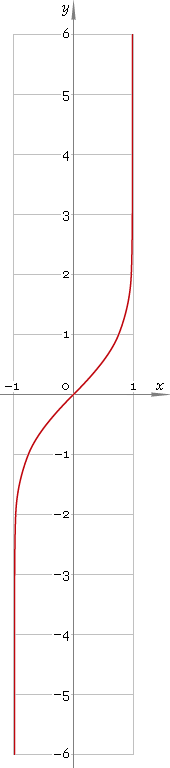The Art of Mathematics

# artanh or arth — arc-hyperbolic tangent function

## 1. Definition

Arc-hyperbolic tangent is inverse of hyperbolic tangent function.

artanhxtanhinvx

With the help of natural logarithm it can be represented as:

artanhx ≡ ln[(1 + x) /(1 − x)] /2

## 2. Graph

Arc-hyperbolic tangent is antisymmetric function defined in the range (−1, 1), points x = ±1 are singular ones. Its graph is depicted below — fig. 1.Fig. 1. Graph of the arc-hyperbolic tangent function y = artanhx.

Function codomain is entire real axis.

## 3. Identities

Property of antisymmetry:

artanh−x = −artanhx

Reciprocal argument:

artanh(1/x) = arcothx

Sum and difference:

artanhx + artanhy = artanh[(x + y) /(1 + xy)]
artanhx − artanhy = artanh[(xy) /(1 − xy)]

## 4. Derivative and indefinite integral

Arc-hyperbolic tangent derivative:

artanh′x = 1 /(1 − x2)

Indefinite integral of the arc-hyperbolic tangent:

∫ artanhx dx = x artanhx + ln(1 − x2) /2 + C

where C is an arbitrary constant.

## 5. How to use

To calculate arc-hyperbolic tangent of the number:

``artanh(−.5);``

To get arc-hyperbolic tangent of the complex number:

``artanh(−.5+i*.4);``

To get arc-hyperbolic tangent of the current result:

``artanh(rslt);``

To get arc-hyperbolic tangent of the number z in calculator memory:

``artanh(mem[z]);``

## 6. Support

Arc-hyperbolic tangent of the real argument is supported in free version of the Librow calculator.

Arc-hyperbolic tangent of the complex argument is supported in professional version of the Librow calculator.